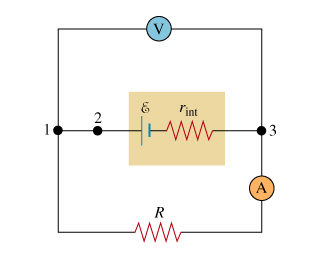###### FREE Expert Solution

Ohm's law:

$\overline{){\mathbf{V}}{\mathbf{=}}{\mathbf{i}}{\mathbf{R}}}$

The power dissipated in DC circuits:

Part A

We are asked to determine the current in the circuit.

Emf of the battery is equal to the sum of potential difference across all resistive elements of the circuit.

ε = irint + iR = i(rint + R)

83% (70 ratings)###### Problem DetailsTo understand how to compute power dissipation in a resistive circuit.

(Figure 1) The circuit in the diagram consists of a battery with EMF E, a resistor with resistance R, an ammeter, and a voltmeter. The voltmeter and the ammeter (labeled V and A) can be considered ideal; that is, their resistances are infinity and zero, respectively. The current in the resistor is I, and the voltage across it is V. The internal resistance of the battery rint is not zero.

Part A. What is the ammeter reading I? Express your answer in terms of ε, R, and rint.

Part B. What is the voltmeter reading V? Express your answer in terms of ε, R, and rint.

In the following parts, you will express the power dissipated in the resistor of resistance R using three different sets of variables.

Part C. What is the power PR dissipated in the resistor? Express your answer in terms of I and V.

Part D. Again, what is the power PR dissipated in the resistor? This time, express your answer in terms of one or more of the following variables: I, rint, and R.

Part E. For the third time, what is the power PR dissipated in the resistor? Express your answer in terms of one or more of the following variables: ε, rint, and R.

Part F. What is the total power Ptotal dissipated in the resistive elements of the circuit? Express your answer in terms of one or more of the following variables: ε, rint, and R.

Part G. What is the total power Ptotal dissipated in the resistive elements of the circuit, in terms of the EMF ε of the battery and the current in the circuit? Express your answer in terms of ε and the ammeter current I.